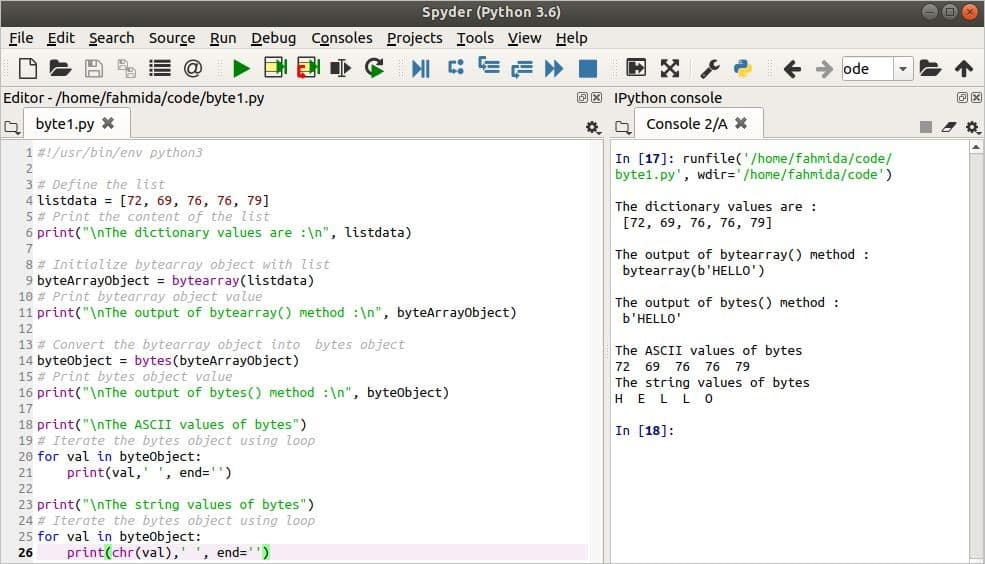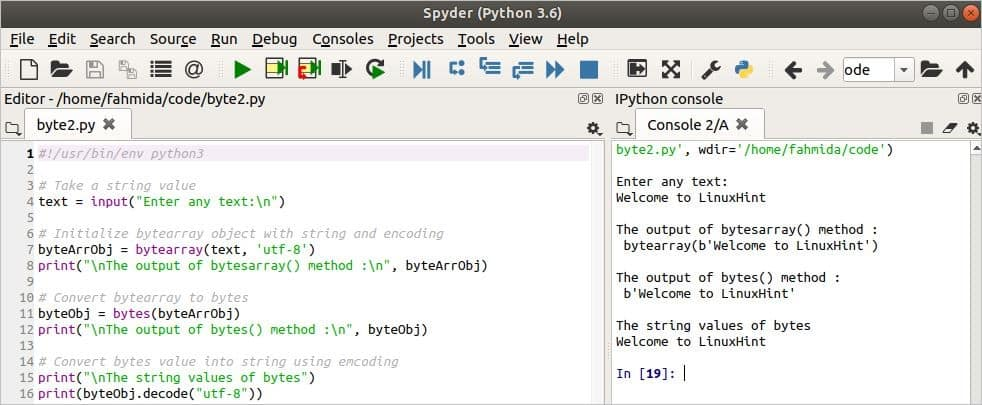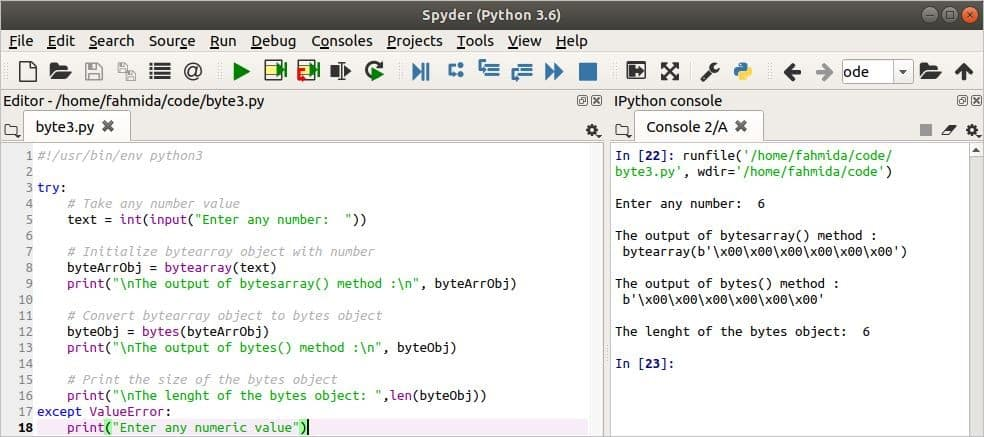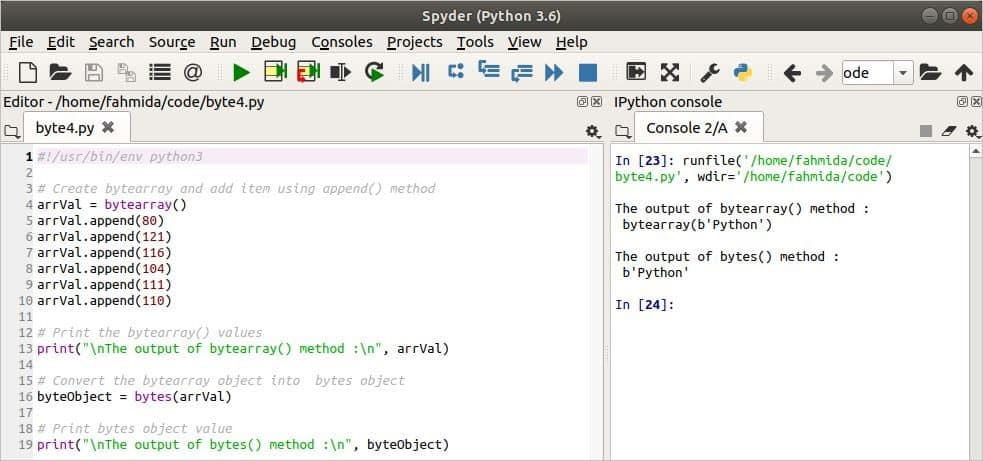Python

# Convert Bytearray to Bytes in Python

Many different types of data objects are supported by Python. Two of them are the objects bytearray and bytes. The bytearray() function returns an array object of bytes. This object is changeable and supports the integer number from 0 to 255. The bytes() function returns bytes objects, is not changeable, and supports the integers from 0 to 255. This article will describe these functions and explain how bytearray objects can be converted into bytes objects.

### Syntax of bytearray() Method

bytearray ([ data_source [, encoding [, errors]]])

The three arguments of this method are optional. The first argument is used to initialize the list of bytes. If the first argument is the string, then the second argument is used for encoding. Finally, the third argument is used to display the error if the encoding fails.

### Syntax of bytes() Method

bytes ([data_source [, encoding [, errors]]])

All arguments of the bytes() function are optional, like the bytearray() method. The functions of these arguments are also the same as the bytearray() method, mentioned above.

The method for converting bytearray to bytes in Python is shown below, using some simple examples for better understanding of this process.

### Example 1: Convert List Data from bytearray to bytes

When the bytearray() function contains only one argument, the value of the argument will be a dictionary datum or variable. The following example shows how a dictionary object can be converted into a bytearray object and how a bytearray object can then be converted into a byte object. Next, the first for loop is used to display the values of the translation table of ASCII codes and the second for loop is used to display the characters of the corresponding ASCII codes.

#!/usr/bin/env python3

# Define the list
listdata = [72, 69, 76, 76, 79]
# Print the content of the list
print("\nThe dictionary values are :\n", listdata)

# Initialize bytearray object with list
byteArrayObject = bytearray(listdata)
# Print bytearray object value
print("\nThe output of bytearray() method :\n", byteArrayObject)

# Convert the bytearray object into  bytes object
byteObject = bytes(byteArrayObject)
# Print bytes object value
print("\nThe output of bytes() method :\n", byteObject)

print("\nThe ASCII values of bytes")
# Iterate the bytes object using loop
for val in byteObject:
print(val,' ', end='')

print("\nThe string values of bytes")
# Iterate the bytes object using loop
for val in byteObject:
print(chr(val),' ', end='')

Output

The following output will appear after running the script. Here, 72, 69, 76, and 79 are the ASCII code of ‘H,’ ‘E,’ ‘L,’ and ‘O,’ respectively.### Example 2: Convert String Data from bytearray to bytes

The following example shows the conversion of bytearray objects to byte objects in string data. Two arguments are used in the bytearray() method of this script. The first argument contains the string value, while the second argument contains the encoding string. Here, ‘utf-8’ encoding is used to convert into a bytearray object. The decode() method is used in the script to convert the bytes objects into string data. The same encoding is used at the time of conversion.

#!/usr/bin/env python3

# Take a string value
text = input("Enter any text:\n")

# Initialize bytearray object with string and encoding
byteArrObj = bytearray(text, 'utf-8')
print("\nThe output of bytesarray() method :\n", byteArrObj)

# Convert bytearray to bytes
byteObj = bytes(byteArrObj)
print("\nThe output of bytes() method :\n", byteObj)

# Convert bytes value into string using emcoding
print("\nThe string values of bytes")
print(byteObj.decode("utf-8"))

Output

The following output will appear after running the script.### Example 3: Convert Integer Data from bytearray to bytes

The previous examples show the conversion of bytearray and bytes based on dictionary and string data. This third example shows the conversion of bytearray into bytes based on the input data. Here, the input value is converted into an integer value and passed as an argument via the bytearray() function, and the bytearray object is then converted into a bytes object. The null values based on the integer number are shown as an output of the bytearray and bytes object. The total number of bytes is counted via the len() method at the end of the script, and will be equal to the integer value passed as an argument into the bytearray() method.

#!/usr/bin/env python3

try:
# Take any number value
text = int(input("Enter any number:  "))

# Initialize bytearray object with number
byteArrObj = bytearray(text)
print("\nThe output of bytesarray() method :\n", byteArrObj)

# Convert bytearray object to bytes object
byteObj = bytes(byteArrObj)
print("\nThe output of bytes() method :\n", byteObj)

# Print the size of the bytes object
print("\nThe lenght of the bytes object: ",len(byteObj))
except ValueError:
print("Enter any numeric value")

Output

After running the script, 6 is taken as input in the following output. The six null values are displayed as the output of bytearray and bytes. When the null values are counted then it displayed 6.### Example 4: Create bytearray Using append() and Convert to bytes

The following example shows how bytearray objects can be created via the append() method and converted into bytes. The arrVal variable is declared here as a bytearray object. Next, the append() method is called six times to add six elements into the array. The ASCII codes of the characters, ‘P,’ ‘y,’ ‘t,’ ‘h,’ ‘o,’ and ‘n,’ are 80, 121, 116, 104, 111 and 1120, respectively. These are added in the bytearray object. This array object is converted into the bytes object later on.

#!/usr/bin/env python3

# Create bytearray and add item using append() method
arrVal = bytearray()
arrVal.append(80)
arrVal.append(121)
arrVal.append(116)
arrVal.append(104)
arrVal.append(111)
arrVal.append(110)

# Print the bytearray() values
print("\nThe output of bytearray() method :\n", arrVal)

# Convert the bytearray object into a bytes object
byteObject = bytes(arrVal)

# Print bytes object value
print("\nThe output of bytes() method :\n", byteObject)

Output

The following output will appear after running the script.### Conclusion

Various methods are shown in this article for converting bytearray to bytes after creating bytearray objects. After reading this article, I hope that you understand the concept of bytearray and bytes, know the way to convert bytearray to bytes, and be able to display the output of bytes as string and characters.